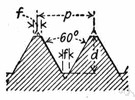# metric function

Also found in: Thesaurus.
ThesaurusAntonymsRelated WordsSynonymsLegend:
 Noun 1metric function - a function of a topological space that gives, for any two points in the space, a value equal to the distance between themmetricmath, mathematics, maths - a science (or group of related sciences) dealing with the logic of quantity and shape and arrangementnatural philosophy, physics - the science of matter and energy and their interactions; "his favorite subject was physics"function, mapping, mathematical function, single-valued function, map - (mathematics) a mathematical relation such that each element of a given set (the domain of the function) is associated with an element of another set (the range of the function)
Based on WordNet 3.0, Farlex clipart collection. © 2003-2012 Princeton University, Farlex Inc.
References in periodicals archive ?
When [beta] [right arrow] 0, the metric function (4) takes the form f(r) = 1 - 2M/r; that is, we get the Schwarzschild black hole geometry.
Since it is the routing metric function to reduce the sum [summation][IRU.sub.l], when using 5MHz channel width, MIC favors to choose links with greater transmission range and that use modulations that transmit smaller number of bits per symbol.
In the literature, to correct the proof of Branciari , some authors assume some superfluous conditions such as Hausdorffness of the induced topology of generalized metric space and continuity of generalized metric function. Inspired by the interesting papers of [3,4] we prove the analog of Banach fixed point theorem in the context of generalized metric space without any further condition.
Assuming, for any path a, its metric is defined by a metric function W(a) and the concatenation of two paths a and b is denoted by a + b, the metric function W(-) is isotonic if W(a) [less than or equal to] W(b) implies both W(a + c) [less than or equal to] W(b + c) and W(c' + a) [less than or equal to] W(c' + b), for all a, b, c, c'.
In this paper, we introduce a partial ordering on dualistic partial metric spaces utilizing partial metric function and use the same to prove a fixed point theorem for single valued nondecreasing mappings on ordered dualistic partial metric spaces.
As we known, a set M associative a function [rho]: M x M [right arrow] [R.sup.+] = {x | x [member of] R, x [greater than or equal to] 0} is called a metric space if for [for all]x, y, z [member of] M, the following conditions for the metric function [rho] hold:
The Heun functions, either general or confluent, are main targets of recent investigations and have been obtained for massless particles evolving in a Universe described by the metric function written as a nonlinear mixture of Schwarzschild, Melvine, and Bertotti-Robinson solutions .
A spherical metric function g(r) in (2) in the asymptotically flat case can have at most two zero points and one minimum between them .
Finsler metric function whose geodesics have two-parameter family of curve in Finsler space is obtained by Matsumoto [27-29].

Site: Follow: Share:
Open / Close